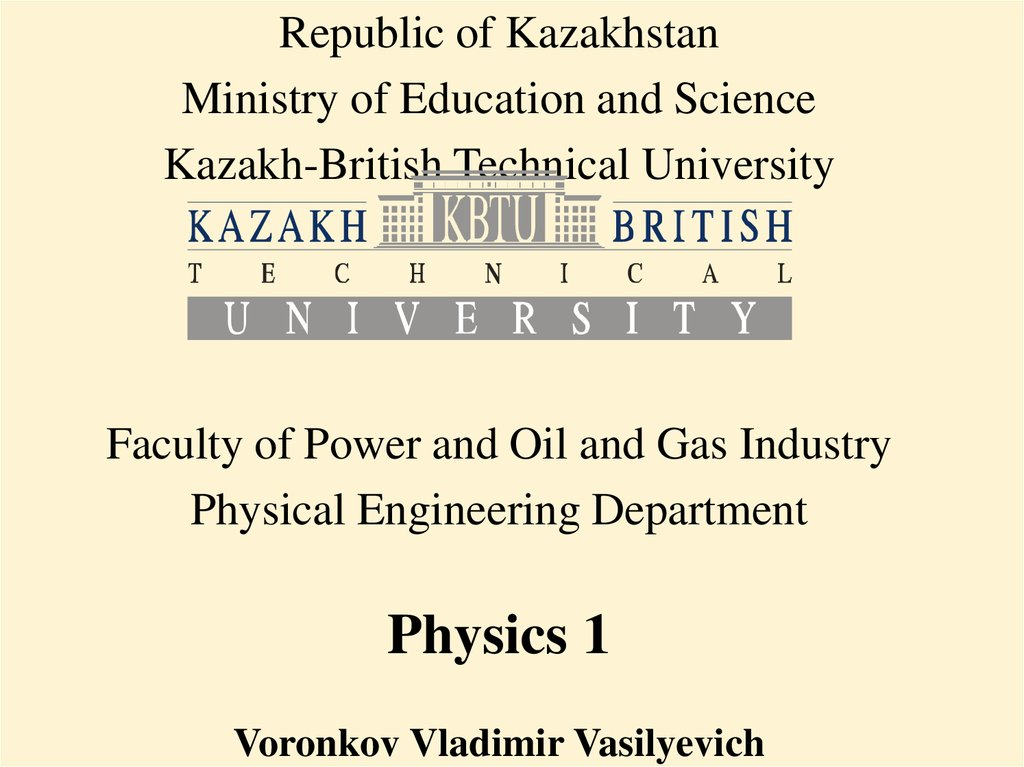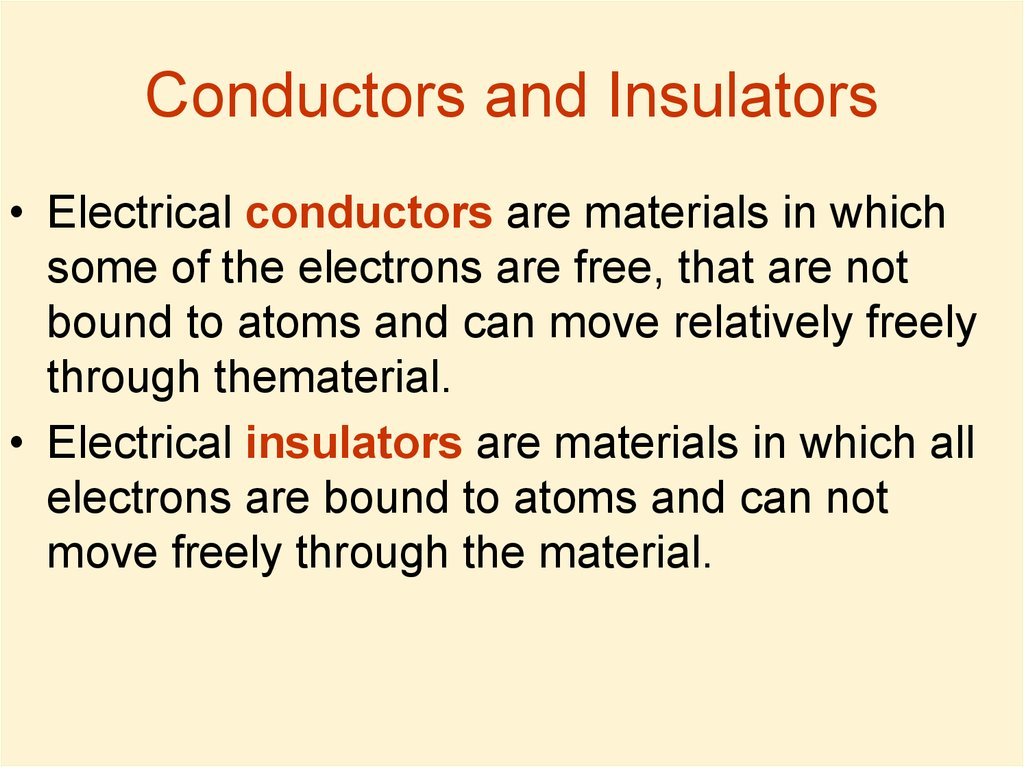# Insulators and Conductors in electric field. Capacitance, Dielectrics. Current, resistance. Electromotive Force

## 1.

Republic of Kazakhstan
Ministry of Education and Science
Kazakh-British Technical University
Faculty of Power and Oil and Gas Industry
Physical Engineering Department
Physics 1

## 2. Lecture 9

Insulators and Conductors in electric field.
Capacitance, Dielectrics.
Current, resistance.
Electromotive Force.

## 3. Conductors and Insulators

• Electrical conductors are materials in which
some of the electrons are free, that are not
bound to atoms and can move relatively freely
through thematerial.
• Electrical insulators are materials in which all
electrons are bound to atoms and can not
move freely through the material.

## 4. Capacitance

• The capacitance C of a capacitor is
defined as the ratio of the magnitude of
the charge on either conductor to the
magnitude of the potential difference
between the conductors:
• Note: net charge of a capacitor is zero. A capacitor
consists of 2 conductors, and Q is the charge on one of
each, and correspondingly –Q is the charge on the other.
• Do not confuse C for capacitance with C for the unit
coulomb.
• Usually V is taken instead of DV for simplicity.

## 5. Parallel – Plate Capacitor

A parallel-plate capacitor
consists of two parallel
conducting plates, each of
area A, separated by a
distance d. When the
capacitor is charged the
plates carry equal
amounts of charge. One
plate carries positive
charge, and the other
carries negative charge.

## 6.

• Using the Gauss theorem we can find that the
value of the electric field between plates is
• The magnitude of the potential difference between
the plates equals:
• And finally:
• So the capacitance of a parallel-plate capacitor is
• Here A is the area of each plate, d is the distance
between plates.

## 8.

• The electric field between the plates of a parallel-plate
capacitor is uniform near the center but nonuniform near
the edges.
• That’s why we applied formula for electric field between
two infinite uniformly charged planes:

• Ceq=C1+C2
• Qnet=Q1+Q2
• V=V1=V2

## 10. Parallel Combination of Capacitors

• The equivalent capacitance of a parallel combination
of capacitors is the algebraic sum of the individual
capacitances and is greater than any of the individual
capacitances.
• Ceq=C1+C2+C3+…
• The total charge on capacitors connected in parallel is
the sum of the charges on the individual capacitors:
• Qnet=Q1+Q2+Q3+…
• The individual potential differences across capacitors
connected in parallel are the same and are equal to
the potential difference applied across the
combination:
• V=V1=V2=V3=…

• Q=Q1=Q2
• V=V1+V2
• 1

## 12. Series Combination of Capacitors

• The charges on capacitors connected in series are the same:
• Q=Q1=Q2=Q3=…
• The total potential difference across any number of capacitors
connected in series is the sum of the potential differences
across the individual capacitors:
• Vnet=V1+V2+V3+…
• The inverse of the equivalent capacitance is the algebraic
sum of the inverses of the individual capacitances and the
equivalent capacitance of a series combination is always less
than any individual capacitance in the combination:

## 13. Capacitors Parallel-Series Combinations:

• Let’s calculate the
equivalent
capacitance step by
step, using the
mentioned above
properties of
capacitors:
• First we merge parallel
capacitors into one:
using that
Cparallel=C1+C2+…

## 15. 2. Joining serial capacitors:

• And finally we
• In circles we have • Then we
join the two
merged capacitors: join series
parallel
conductors:
conductors into
one:

## 16. Energy Stored in a Charged Capacitor

If a capacitor has charge Q then it’s difference
of potentials V is V=Q/C, then the work dW,
necessary to transfer small charge dq from one
capacitor’s conductor to another is:
Then the total work required to charge the
capacitor from q = 0 to final charge q = Q is

## 17. Energy Stored in a Charged Capacitor

• The work done in charging the capacitor
appears as electric potential energy U stored in
the capacitor
• Here U is the energy, stored in the capacitor,
DV – difference of potentials on the capacitor
• This result applies to any capacitor, regardless
of its geometry.

## 18. Energy in a Capacitor

Usually V is used instead of DV for the
difference of potentials, then the expressions
for energy, stored in a capacitor is:

## 19. Energy in Electric Fields

• Let’s take a parallel-plate capacitor:
• V - the potential difference between the
plates of a capacitor,
• d - distance between the plates,
• A – the area of each plate,
• E - the electric field between the plates of
a capacitor. Then V=Ed.
• Then the energy of the electric field in the
capacitor is:

## 20. Energy density of Electric Field

• The volume, occupied by the electric field
is Ad, then the energy density of the
electric field is:
• The energy density in any electric field is
proportional to the square of the
magnitude of the electric field at a given
point.

## 21. Dielectrics

• Many materials (like paper, rubber, plastics,
glass …) do not conduct electricity easily – we
call them insulators.
• But they modify the electric field they are placed
in, that’s why they are called dielectrics.
• E0 – the electric field without the dielectric
• E – the electric field in the presence of the
dielectric
• k – the dielectric constant

## 22. Dielectric strength

• The dielectric strength equals the
maximum electric field that can exist in a
dielectric without electrical breakdown.
Note that these values depend strongly on
the presence of impurities and flaws in the
materials.

## 24. Atomic Description of Dielectrics

• Dielectric can be made up of
polar molecules. The dipoles
are randomly oriented in the
absence of an electric field.
• When an external Electric
field is applied, its molecules
partially align with the field.
Now the dielectric is
polarized.

## 25. Polar and Nonpolar molecules of Dielectric

• The molecules of the dielectric can be polar or
nonpolar.
• The case of polar molecules are considered in the
previous slide.
• If the molecules of the dielectric are nonpolar then the
electric field produces some charge separation in
every molecule of the dielectric, and an induced dipole
moment is created. These induced dipole moments
tend to align with the external field, and the dielectric
is polarized.
• Thus, we can polarize a dielectric with an external field
regardless of whether the molecules are polar or
nonpolar.

## 26. Dielectric polarization

• The degree of alignment of the molecules
with the electric field depends on
temperature and on the magnitude of the
electric field.
• In general, the alignment increases with
decreasing temperature and with
increasing electric field.

## 27. Induced Electric field in Dielectric

• When an external field E0
is applied, a torque is
exerted on the dipoles,
causing them to partially
align with the field.
• That’s why dielectric’s
molecules produces
induced electric field Eind,
opposite to the external E0.

## 28. Capacitor with Dielectric

• So the electric field is k times less in a capacitor
with a dielectric, its dielectric constant is k:
• Then, the potential difference is k times less:
without dielectric:
V0 =E0d
with dielectric:
V=Ed= E0d/k.
V=V0/k.

## 29.

• As the charge Q on the capacitor is not
changed:
• C0=Q/V0,
V=V0/k
• C=Q/V=kC0V0/V0=kC0
C=kC0
• So the capacitance increases in k if a
dielectric completely fills the distance
between the plates of a capacitor.

## 30. Usage of Dielectrics in Capacitors

• Insulating materials have k>1 and dielectric
strength greater than that of air, so usage
– Increase in capacitance.
– Increase in maximum operating voltage.
– Possible mechanical support between the
plates, which allows the plates to be close
together without touching, thereby decreasing
d and increasing C.

## 31. Electric Current

• Electric current (or just current) is defined as the total
charge that passes through a given cross-sectional area
per unit time.
• Current can be composed of
– moving negative charges such as electrons or negatively
charged ions;
– moving positive charges such as protons or positively charged
ions.
DQ - the amount of charge passing through the cross
sectional area of a wire
DT - a time interval of the passing.
The average current is:
• The instantaneous current is:

## 32. Current direction

• By convention the direction of the current
is the direction of positive charges would
move.

## 33. Ohm’s Law

• Ohm’s law states that
• For many materials the resistance is
constant over a wide range of potential
differences:
V = IR.
• Resistance is defined as the opposition to
the flow of electric charge.

## 34. Electromotive Force

• A device with the ability to maintain potential
difference between two points is called a
source of electromotive force (emf). The
most familiar sources of emf are batteries and
generators.
• Batteries convert chemical energy into electric
energy.
• Generators transforms mechanical energy into
electric energy.
• Since emf is work per unit charge, it is
expressed in the same unit as potential
difference: the joule per coulomb, or volt.

## 35. Units in Si

Capacitance
Current
Resistance
Electro motive force (emf)
Energy density
C
I
R
e
uE
F=C/V
A=C/s
Ohm=V/A
V
J/m3=kg/(m*s2)